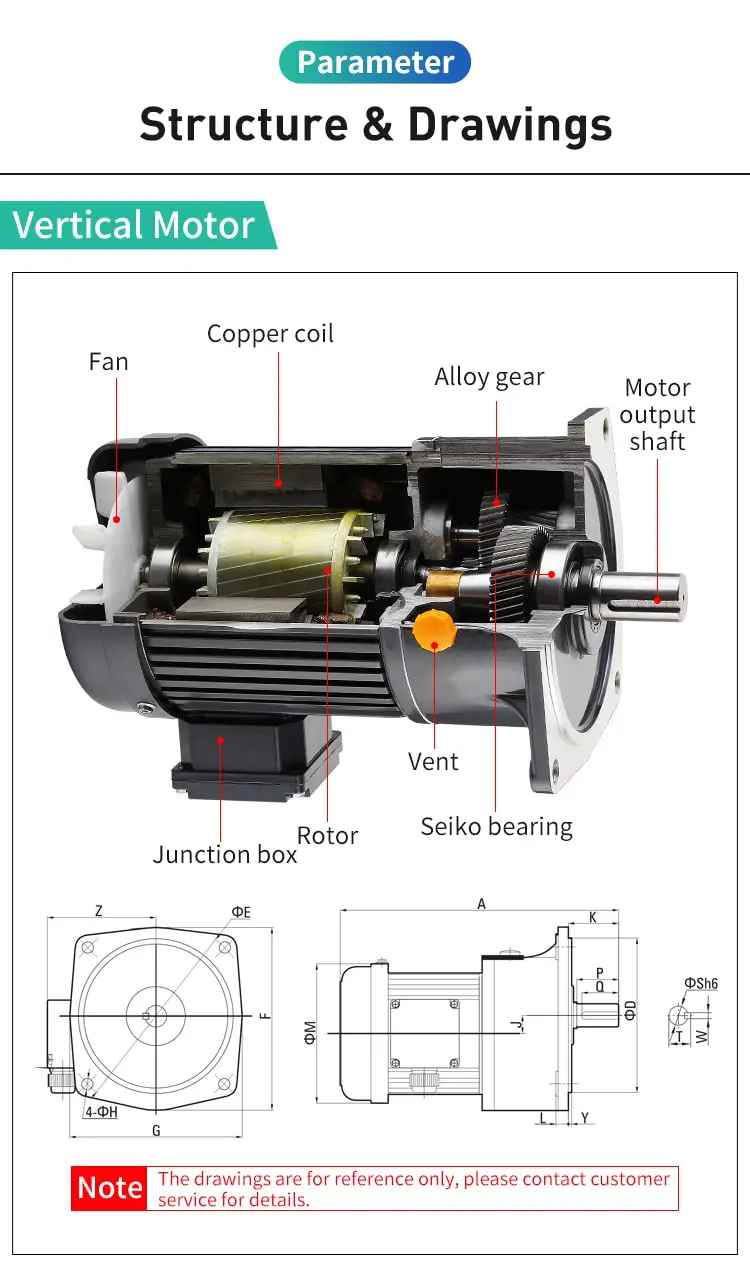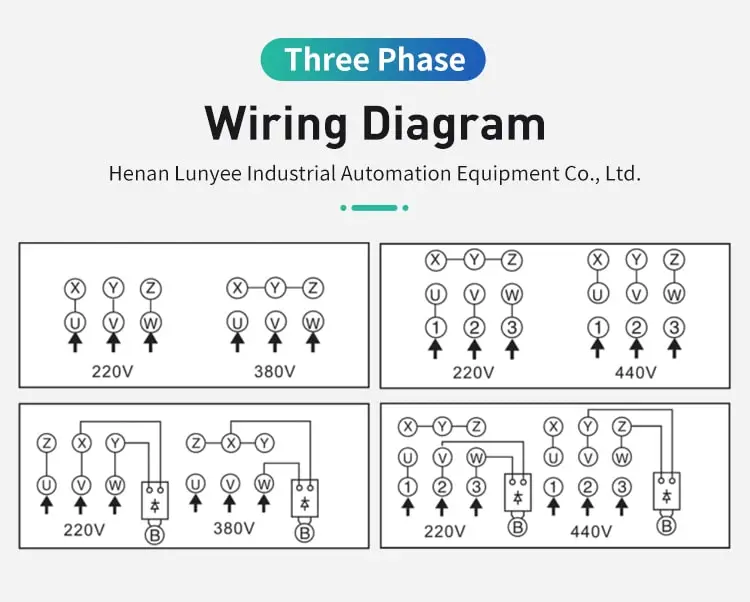By Jasmine 2023-02-27 17:30:09

#### How to calculate rotor resistance of induction motor?

Two common methods to calculate the rotor resistance of an induction motor are direct measurement using a No-load or Blocked Rotor Test, or using an approximation formula Rotor Resistance = (Stator Voltage / Stator Current) - (Xs).

Calculating the rotor resistance of an induction motor is an important step in determining its performance and behavior under different operating conditions. The rotor resistance, also known as the rotor impedance, is a measure of the resistance and reactance of the rotor circuit. It is an important parameter in the analysis and design of induction motors, as it affects the starting characteristics, running behavior, and efficiency of the motor.

To calculate the rotor resistance of an induction motor, we need to know the following values:

1. Rotor winding resistance: This is the DC resistance of the rotor winding, which is determined by measuring the resistance of the rotor winding using a digital multimeter or a rotor tester.

2. Rotor slip: This is the difference between the synchronous speed of the rotor and the actual speed of the rotor, expressed as a percentage. It is a measure of the relative motion between the rotor and the stator magnetic field, and is an important parameter in the analysis of induction motors.

3. Synchronous reactance of the rotor: This is the reactance of the rotor circuit at the synchronous speed, and is determined by measuring the impedance of the rotor winding at the synchronous speed using a rotor tester or an equivalent circuit model of the motor.

Once we have these values, we can use the following formula to calculate the rotor resistance of the induction motor:

Rotor resistance (Ohms) = Rotor winding resistance / (1 - Rotor slip)

For example, consider an induction motor with a rotor winding resistance of 0.5 Ohms and a rotor slip of 5%. Using the formula above, we can calculate the rotor resistance of the motor as follows:

Rotor resistance (Ohms) = 0.5 Ohms / (1 - 0.05) = 0.525 Ohms

It is important to note that the rotor resistance calculated using the above formula is the DC resistance of the rotor circuit. In order to account for the effect of the rotor reactance on the performance of the motor, we need to calculate the complex rotor impedance, which is given by the following formula:

Complex rotor impedance (Ohms) = Rotor resistance + j (Synchronous reactance of the rotor)

where j is the imaginary unit.

For example, consider the same induction motor with a rotor winding resistance of 0.5 Ohms, a rotor slip of 5%, and a synchronous reactance of the rotor of 0.3 Ohms. Using the formula above, we can calculate the complex rotor impedance of the motor as follows:

Complex rotor impedance (Ohms) = 0.5 Ohms + j (0.3 Ohms) = 0.5 + j0.3 Ohms

In conclusion, calculating the rotor resistance of an induction motor is an important step in understanding its performance and behavior under different operating conditions. It is determined using the rotor winding resistance, rotor slip, and synchronous reactance of the rotor, and is used to calculate the complex rotor impedance, which accounts for the effect of the rotor reactance on the performance of the motor.